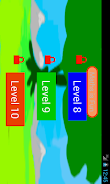3.4
 Package: com.Maths.fifthgradegooglelite Version: 3.0 (3) Size: 3.4 MB Update on: December 03, 2013 Installs: 50,000 Signature: f5d1c05ff6a1c94136b2c770fa8d97f0cd825863 APK File SHA1: 64e2e7814e9d51b93d08d21ce51c5a69b8d39893

Searching for fifth grade learning games? You are at the right place.
Fifth grade mathematics tests (Infinite numbers - System generated questions and answers,test, questions are Different every time) for 5th grade students.
This Fifth grade learning games contains syllabus based on Common Core math. 5th grade math games free contains 4 Levels (Only one level in
Lite and all levels in Pro).

► 5.OA 5th grade math Numerical expressions worksheets
► 5.OA 5th grade math Patterns worksheets
► 5.NBT 5th grade math Place value and comparison worksheets
► 5.NBT.A.2 5th grade math Powers of 10 worksheets
► 5.NBT.A.3 5th grade math Decimal comparison worksheets
► 5.NBT.A.4 5th grade math Round Decimals worksheets
► 5.NBT 5th grade math Multiplication worksheets
► 5.NBT 5th grade math Division worksheets
► 5.NBT 5th grade math Decimal Subtraction flash cards
► 5.NBT 5th grade math Decimal Multiplication table worksheets
► 5.NBT 5th grade math Decimal Division worksheets
► 5.NF 5th grade math Fraction - addition - mixed numbers worksheets
► 5.NF 5th grade math Fraction - Subtraction worksheets
► 5.NF 5th grade math Fraction - subtraction - mixed numbers worksheets
► 5.NF 5th grade math Fraction - multiplication worksheets
► 5.NF 5th grade math Fraction - Division worksheets
► 5.MD 5th grade math Measurement and data worksheets
► 5.G 5th grade math Geometry-1
► 5.G 5th grade math Graph
► 5.G 5th grade math Geometry-2

Fifth grade maths common core syllabus - 5th grade math activities / worksheets :
5.OA Operations and Algebraic Thinking
► Evaluate Numerical expressions
► Write simple numerical expressions
► Analyze numerical patterns and relationships.

5th grade math common core syllabus 5.NBT Number and Operations in Base Ten with Worksheets / activities
► place value for 5th graders to understand properties of operations to perform multi-digit arithmetic in base ten
► add and subtract - place value, properties of operations, relationship between addition and subtraction in base ten
► Patterns, Read, write, and compare decimals to thousandths
► Place value understanding to round decimals to any place
► Relationship between multiplication and division

5th grade math Worksheets / tests / quiz / activities - CBSE 5.NF Number and Operations Fractions
► fractions as numbers for 5th grade
► Add and subtract fractions with unlike denominators
► Solve word problems involving addition and subtraction of fractions
► Interpret a fraction as division of the numerator by the denominator
► multiply a fraction or whole number by a fraction
► area of a rectangle with fractional side lengths
► Compare two fractions-same & different numerator /denominator
► multiply a fraction by a whole number
► Compare two decimals to hundredths

Worksheets - Fifth grade math activities- common core syllabus 5.MD Measurement and Data
► conversion measurement units within a given measurement system
► Line plot

Worksheets / tests / quiz / math games covers 5.G Geometry
► Graph points on the coordinate plane to solve real-world and mathematical problems.
► Use a pair of perpendicular number lines called axes to define coordinate systems

This Fifth grade learning games free app, learn Mathematics wherever you are. 5th grade math testing preparation helps your kid.
This kids math games for Fifth grade is useful for school students of second grade, 5th grade and Fifth grade to learn and test their understanding in one go.
•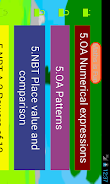•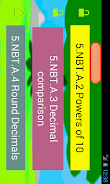•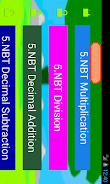•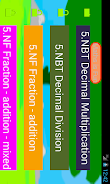•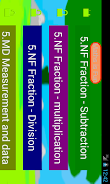•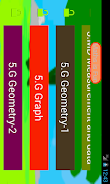•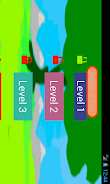•